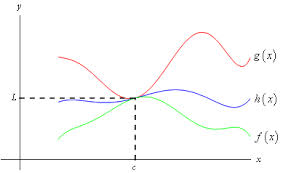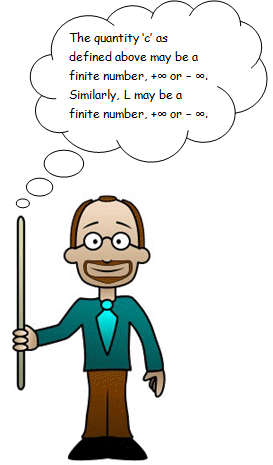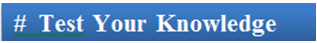×#### Thank you for registering.

One of our academic counsellors will contact you within 1 working day.

Click to Chat

1800-1023-196

+91-120-4616500

CART 0

• 0

MY CART (5)

Use Coupon: CART20 and get 20% off on all online Study Material

ITEM
DETAILS
MRP
DISCOUNT
FINAL PRICE
Total Price: Rs.

There are no items in this cart.
Continue Shopping```Limits using Sandwich Theorem and L’Hospital’s Rule

Table of Content

Sandwich Theorem

We now discuss certain illustrations involving greatest integer functions

L’Hospital’s Rule

Related Resources

Sandwich TheoremSandwich theorem is an important concept of limits. It is often termed as the Squeeze theorem, Pinching Theorem or the Squeeze Lemma. The Squeeze principle is generally used on limit problems where the usual algebraic methods like the algebraic or the factorization methods fail in computing limits. Hence, in such a case the sandwich or the squeeze theorem tries to squeeze our problem in between the limits of two simple functions whose limits can be evaluated with ease and are in fact equal. In fact, this is the reason behind the name of this theorem.

Let f(x), g(x) and h(x) be three real numbers having a common domain D such that f(x) ≤ h(x) ≤ g(x) ∀ x in some interval containing the point c. Then,

If lim x→a f(x) = lim x→a g(x) = l, then lim x→a h(x) = l.

This is known as Sandwich Theorem.Illustration:

Examine lim x→0 √(1 – cos x)/x

Solution:View the following video for more on Sandwich Theorem

We now discuss certain illustrations involving greatest integer functions

Illustration 1:

lim x→3 ([x] – 3)/(x – 3)

Solution:

lim x→3 ([x] – 3)/(x – 3)

Towards the right of x = 3, [x] = 3

⇒ [x] – 3 = 0, in the right neighborhood of x = 3

⇒ lim x→3+0 ([x] – 3)/(x – 3) = 0

Towards the left of x = 3, [x] = 2

⇒ [x] – 3 = -1, in the left neighborhood of x =3

⇒ lim x→3+0 ([x] - 3)/(x – 3) = lim x→3+0 (-1)/(x – 3) = ∞.

Thus lim x→3 ([x] – 3)/(x – 3) does not exist.

__________________________________________________________________________

Illustration 2:

Prove that lim n→∞ x5 [1/x3] = 0, where [.] denotes the greatest integer function.

Solution:

We know that (x - 1) < |x| ≤ x ∀ x ∈ R

So, 1/x3 -1 < [1/x3] ≤ 1/x3

⇒ x5 (1/x3 -1) < x5 [1/x3 ] ≤ x5 (1/x3 ) for x > 0

And lim x→0  x2 - x5 = lim x→0 x2 = 0

⇒ lim x→0+  x5 [1/x3 ] = 0

Also, x5 (1/x3) ≤ x5 [1/x3 ] < x5 (1/x3 -1) for x < 0

⇒ lim x→0-  x5 [1/x3] = 0   => limx→0  x5 [1/x3 ] = 0.

__________________________________________________________________________

Illustration 3:

Evaluate lim n→∞ ([x] + [2x] + [3x] + ...........= [nx]) / n2, where [.] denotes the greatest integer function.

Solution:

We know that (x - 1) < x

⇒ x + 2x + .......... + nx – n < ∑r=1n [rx] ≤ x + 2x +  ......... + nx

⇒ (x.{n (n + 1)/2} - n)  < ∑r=1n [rx] ≤ {x.n (n + 1)/2}

⇒ x/2 (1 + 1/n) – 1/n < 1/n2 ∑r=1n [rx] ≤ x/2 (1 + 1/n)

Now, lim n→∞ x/2 (1 + 1/n) = x/2 and lim n→∞ x/2 (1 + 1/n) – 1/n = x/2.

Using Sandwich theorem we find that lim n→∞ ([x] + [2x] +  ..........+ [nx])/n2 = x/2

L’Hospital’s RuleThe L Hospital’s Rule is used in cases where the limit obtained is in the form of 0/0, ∞/∞, ∞ – ∞, 0, ∞, 00, ∞0, 1∞. The other indeterminate forms are ∞-∞,0,∞,00,∞0,1∞. These are known as indeterminate forms. According to this rule,

Let f(x) and g(x) be functions differentiable in the neighborhood of the point a, except may be at the point a itself. If lim x→a f(x) = 0 = lim x→a g(x) or lim x→a f(x) = ∞ = lim x→a g(x), then

lim x→a f(x)/g(x) = lim x→a f ’(x)/g'(x) provided that the limit on the right either exists as a finite number or is ± ∞ .

The following illustrations describe the rule:

Illustration 1:

Evaluate  lim x→1 (1 – x + ln x)/(1 + cos πx)

Solution:

lim x→1 (1 – x + ln x)/(1 + cos πx) (of the form 0/0)

= lim x→1 (1 – 1/x)/(-π sin πx) (still of the form 0/0)

=  lim x→1 (x – 1)/(πx sin πx) (algebraic simplification)

=  lim x→1 1/(πx sin πx + π2x cos πx ) (L' Hospital's rule again)

= - 1/π2

_________________________________________________________________________________________

Illustration 2:

Let f : R→R be such that f(1) = 3 and f’(1) = 6. Then lim x→0 f(1+x)/f(1))1/x equals (IIT JEE 2002)

(a) 1                                                                              (b) e1/2

(c) e3                                                                            (d) e2

Solution:

The given function is y = [f(1 + x)/ f(1)]1/x

Taking log both sides we have,

log y = 1/x [log f(1 + x) – log f(1)]

Taking limit on both sides we get,

lim x→0 log y = lim x→0 [f’(1 + x) / f(1 + x)]  (using Hospital’s rule)

= f’(1)/ f(1)

= 6/3

log (lim x→0 y) = 2.

lim x→0 y = e2 .

______________________________________________________________________

Illustration 3:

Evaluate lim x→y  (xy - yx)/(xx - yy).

Solution:

lim x→y  (xy - yx)/(xx - yy) which is a [0/0] form

= lim x→y (yxy-1 - yx log y)/(xx log (ex))

= (1 – log y)/ log (ey)?Q1. In Sandwich theorem, the function f(x) ≤ h(x) ≤ g(x) ∀ x in some interval containing the point c. Then,

(a) c may be a finite number, +∞ or – ∞.

(b) c can only be a finite number

(c) c has to be +∞ or – ∞.

(d) none of these.

Q2. L’Hospital’s Rule can be used in solving limits

(a) c may be a finite number, +∞ or – ∞.

(b) of indeterminate forms.

(c) c has to be +∞ or – ∞.

(d) none of these.

Q3. The limit L as obtained in Sandwich theorem

(a) L has to be positive

(b) L can only be a finite number

(c) L has to be +∞ or – ∞.

(d) L may be a finite number, +∞ or – ∞.

Q4. Using L’Hospital’s Rule, we have lim x→1 [f(x)/g(x)] =

(a) f(x)/g’(x)

(b) f’(x)/g(x)

(c) f’(x)/g’(x)

(d) none of these.Q1.

Q2.

Q3.

Q4.

(a)

(b)

(d)

(c)

Related Resources

You may wish to refer Differentiability.

For getting an idea of the type of questions asked, refer the previous year papers.

To read more, Buy study materials of Limtis and Continuity comprising study notes, revision notes, video lectures, previous year solved questions etc. Also browse for more study materials on Mathematics here.
```### Course Features

• 731 Video Lectures
• Revision Notes
• Previous Year Papers
• Mind Map
• Study Planner
• NCERT Solutions
• Discussion Forum
• Test paper with Video Solution